## 22.1 Longitudinal Ordinal Models as Unifying Concepts

This material in this section is taken from hbiostat.org/talks/rcteff.html. See also hbiostat.org/proj/covid19/ordmarkov.html

### 22.1.1 General Outcome Attributes

• Timing and severity of outcomes
• Handle
• terminal events (death)
• non-terminal events (MI, stroke)
• recurrent events (hospitalization)
• Break the ties; the more levels of Y the better: fharrell.com/post/ordinal-info
• Maximum power when there is only one patient at each level (continuous Y)

### 22.1.2 What is a Fundamental Outcome Assessment?

• In a given week or day what is the severity of the worst thing that happened to the patient?
• Expert clinician consensus of outcome ranks
• Spacing of outcome categories irrelevant
• Avoids defining additive weights for multiple events on same week
• Events can be graded & can code common co-occurring events as worse event
• Can translate an ordinal longitudinal model to obtain a variety of estimates
• time until a condition
• expected time in state
• Bayesian partial proportional odds model can compute the probability that the treatment affects mortality differently than it affects nonfatal outcomes
• Model also elegantly handles partial information: at each day/week the ordinal Y can be left, right, or interval censored when a range of the scale was not measured

### 22.1.3 Examples of Longitudinal Ordinal Outcomes

• censored at 3w: 000
• death at 2w: 01
• longitudinal binary logistic model OR $$\approx$$ HR
• 0=at home 1=hospitalized 2=MI 3=dead
• hospitalized at 3w, rehosp at 7w, MI at 8w & stays in hosp, f/u ends at 10w: 0010001211
• 0-6 QOL excellent–poor, 7=MI 8=stroke 9=dead
• QOL varies, not assessed in 3w but pt event free, stroke at 8w, death 9w: 12[0-6]334589
• MI status unknown at 7w: 12[0-6]334[5-7]891
• Can make first 200 levels be a continuous response variable and the remaining values represent clinical event overrides
• 1 Better: treat the outcome as being in one of two non-contiguous values {5,7} instead of [5-7] but no software is currently available for this

• ### 22.1.4 Statistical Model

• Proportional odds ordinal logistic model with covariate adjustment
• Patient random effects (intercepts) handle intra-patient correlation
• Better fitting: Markov model
• handles absorbing states, extremely high day-to-day correlations within subject
• faster, flexible, uses standard software
• state transition probabilities
• after fit translate to unconditional state occupancy probabilities
• use these to estimate expected time in a set of states (e.g., on ventilator or dead); restricted mean survival time without assuming PH
• Extension of binary logistic model
• Generalization of Wilcoxon-Mann-Whitney Two-Sample Test
• No assumption about Y distribution for a given patient type
• Does not use the numeric Y codes

## 22.2 Case Studies

• Random effects model for continuous Y: Section 7.8.3
• Markov model for continuous Y: Section 7.8.4
• Multiple detailed case studies for discrete ordinal Y: hbiostat.org/proj/covid19.
• ORCHID: hydroxychloroquine for treatment of COVID-19 with patient assessment on select days
• VIOLET: vitamin D for serious respiratory illness with assessment on 28 consecutive days
• Large power gain demonstrated over time to recovery or ordinal status at a given day
• Loosely speaking serial assessments for each 5 day period had the same statistical information as a new patient assessed once
• ACTT-1: NIH-NIAID Remdesivir study for treatment of COVID-19 with daily assessment while in hospital, select days after that with interval censoring
• Assesses time-varying effect of remdesivir
• Handles death explicitly, unlike per-patient time to recovery
• Other: Bayesian and frequentist power simulation, exploration of unequal time gaps, etc.

## 22.3 Case Study For 4-Level Ordinal Longitudinal Outcome

• VIOLET: randomized clinical trial of seriously ill adults in ICUs to study the effectiveness of vitamin D vs. placebo
• Daily ordinal outcomes assessed for 28 days with very little missing data
• Original paper: DOI:10.1056/NEJMoa1911124 focused on 1078 patients with confirmed baseline vitamin D deficiency
• Focus on 1352 of the original 1360 randomized patients
• Extensive re-analyses:
• Fitted a frequentist first-order Markov partial proportional odds (PO) model to 1352 VIOLET patients using the R VGAM package to simulate 250,000 patient longitudinal records with daily assessments up to 28d: hbiostat.org/data/repo/simlongord.html
• Simulation inserted an odds ratio of 0.75 for tx=1 : tx=0 (log OR = -0.288)
• Case study uses the first 500 simulated patients
• 13203 records
• average of 26.4 records per patient out of a maximum of 28, due to deaths
• full 250,00 and 500-patient datasets available at hbiostat.org/data
• 4-level outcomes:
• patient at home
• in hospital or other health facility
• on ventilator or diagnosed with acute respiratory distress syndrome (ARDS)
• Death is an absorbing state
• only possible previous states are the first 3
• at baseline no one was at home
• a patient who dies has Dead as the status on their final record, with no “deaths carried forward”
• later we will carry deaths forward just to be able to look at empirical state occupancy probabilities (SOPs) vs. model estimates
• Frequentist modeling using the VGAM package allows us to use the unconstrained partial PO (PPO) model with regard to time, but does not allow us to compute uncertainty intervals for derived parameters (e.g., SOPs and mean time in states)
• Can use the bootstrap to obtain approximate confidence limits (as below)
• Bayesian analysis using the rmsb package provides exact uncertainty intervals for derived parameters but at present rmsb only implements the constrained PPO model when getting predicted values
• PPO for time allows mix of outcomes to change over time (which occurred in the real data)
• Model specification:
• For day $$t$$ let $$Y(t)$$ denote the ordinal outcome for a patient
$$\Pr(Y \geq y | X, Y(t-1)) = \text{expit}(\alpha_{y} + X\beta + g(Y(t-1), t))$$

• $$g$$ contains regression coefficients for the previous state $$Y(t-1)$$ effect, the absolute time $$t$$ effect, and any $$y$$-dependency on the $$t$$ effect (non-PO for $$t$$)

• Baseline covariates: age, SOFA score (a measure of organ function), treatment (tx)

• Time-dependent covariate: previous state (yprev, 3 levels)

• Time trend: linear spline with knot at day 2 (handles exception at day 1 when almost no one was sent home)

• Changing mix of outcomes over time

• effect of time on transition ORs for different cutoffs of Y
• 2 time components (one slope change) $$\times$$ 3 Y cutoffs = 6 parameters related to day
• Reverse coding of Y so that higher levels are worse

### 22.3.1 Descriptives

• all state transitions from one day to the next
• SOPs estimated by proportions (need to carry death forward)
Code
require(rms)
require(data.table)
require(VGAM)
getHdata(simlongord500)
d <- simlongord500
setDT(d, key='id')
d[, y     := factor(y,     levels=rev(levels(y    )))]
d[, yprev := factor(yprev, levels=rev(levels(yprev)))]
setnames(d, 'time', 'day')
# Show descriptive statistics for baseline data
html(describe(d[day == 1, .(yprev, age, sofa, tx)], 'Baseline Variables'))
Baseline Variables Descriptives
Baseline Variables

4 Variables   500 Observations

yprev
nmissingdistinct
50002
 Value      In Hospital/Facility            Vent/ARDS
Frequency                   340                  160
Proportion                 0.68                 0.32


age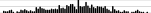nmissingdistinctInfoMeanGmd.05.10.25.50.75.90.95
5000730.99956.0817.4928.9536.0046.0058.0067.0075.1079.05
lowest : 19 20 21 22 23 , highest: 89 90 91 92 94
sofa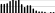nmissingdistinctInfoMeanGmd.05.10.25.50.75.90.95
5000180.9925.2743.901 0.00 1.00 3.00 5.00 7.2510.0011.00
lowest : 0 1 2 3 4 , highest: 13 14 15 17 18
 Value          0     1     2     3     4     5     6     7     8     9    10    11
Frequency     38    38    41    51    59    53    58    37    24    32    32    17
Proportion 0.076 0.076 0.082 0.102 0.118 0.106 0.116 0.074 0.048 0.064 0.064 0.034

Value         12    13    14    15    17    18
Frequency      8     4     4     2     1     1
Proportion 0.016 0.008 0.008 0.004 0.002 0.002


tx
nmissingdistinctInfoSumMeanGmd
500020.752560.5120.5007

Code
# Check that death can only occur on the last day
d[, .(ddif=if(any(y == 'Dead')) min(day[y == 'Dead']) -
max(day) else NA_integer_),
by=id][, table(ddif)]

ddif 0 51

Code
propsTrans(y ~ day + id, data=d, maxsize=4, arrow='->') +
theme(axis.text.x=element_text(angle=90, hjust=1))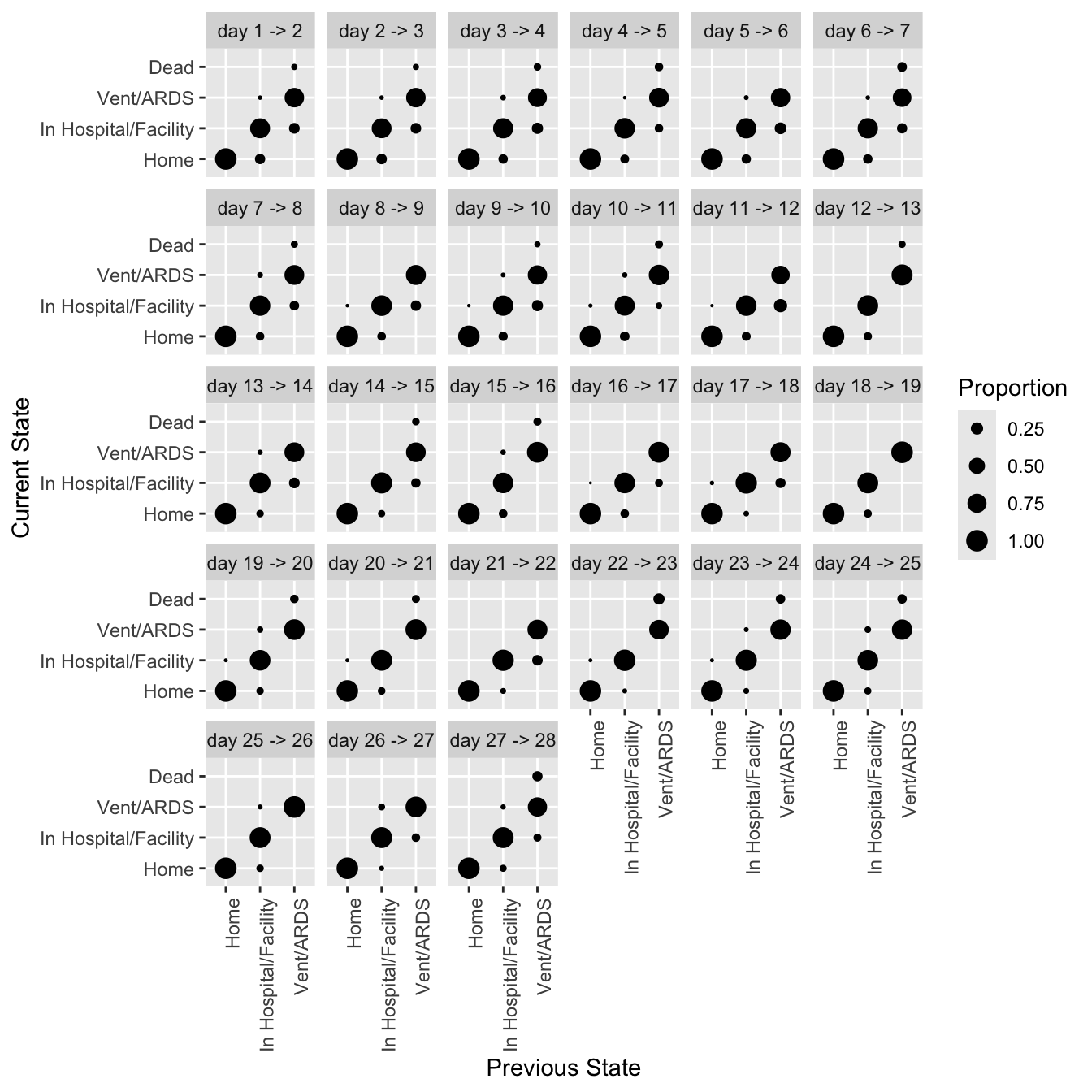Figure 22.1: Transition proportions from data simulated from VIOLET

Show state occupancy proportions by creating a data table with death carried forward.

Code
w <- d[day < 28 & y == 'Dead', ]
w[, if(.N > 1) stop('Error: more than one death record'), by=id]
Empty data.table (0 rows and 1 cols): id
Code
w <- w[, .(day = (day + 1) : 28, y = y, tx=tx), by=id]
u <- rbind(d, w, fill=TRUE)
setkey(u, id)
u[, Tx := paste0('tx=', tx)]
propsPO(y ~ day + Tx, data=u) +
guides(fill=guide_legend(title='Status')) +
theme(legend.position='bottom', axis.text.x=element_text(angle=90, hjust=1))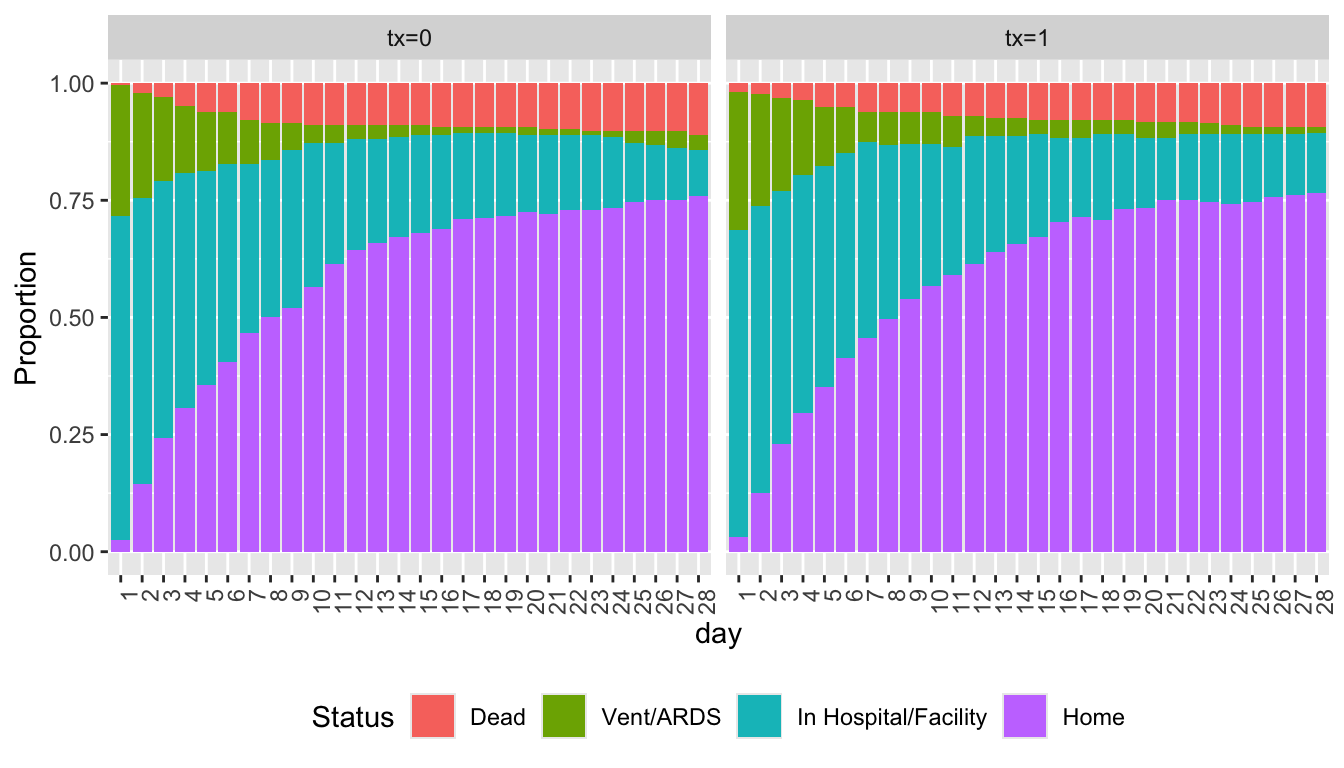Figure 22.2: State occupancy proportions from simulated VIOLET data with death carried forward

### 22.3.2 Model Fitting

• Fit the PPO first-order Markov model without assuming PO for the time effect
• Also fit a model that has linear splines with more knots to add flexibility in how time and baseline covariates are transformed
• Disregard the terrible statistical practice of using asterisks to denote “significant” results
Code
f <- vglm(ordered(y) ~ yprev + lsp(day, 2) + age + sofa + tx,
cumulative(reverse=TRUE, parallel=FALSE ~ lsp(day, 2)), data=d)
summary(f)

Call:
vglm(formula = ordered(y) ~ yprev + lsp(day, 2) + age + sofa +
tx, family = cumulative(reverse = TRUE, parallel = FALSE ~
lsp(day, 2)), data = d)

Coefficients:
Estimate Std. Error z value Pr(>|z|)
(Intercept):1              -5.02973    0.62819  -8.007 1.18e-15 ***
(Intercept):2             -13.19283    0.57656 -22.882  < 2e-16 ***
(Intercept):3             -19.57982    0.93523 -20.936  < 2e-16 ***
yprevIn Hospital/Facility   8.77312    0.26552  33.042  < 2e-16 ***
yprevVent/ARDS             15.13834    0.32138  47.104  < 2e-16 ***
lsp(day, 2)day:1           -1.43145    0.28423  -5.036 4.75e-07 ***
lsp(day, 2)day:2           -0.70738    0.25654  -2.757  0.00583 **
lsp(day, 2)day:3            0.13745    0.46781   0.294  0.76890
lsp(day, 2)day':1           1.48443    0.28596   5.191 2.09e-07 ***
lsp(day, 2)day':2           0.74601    0.26061   2.863  0.00420 **
lsp(day, 2)day':3          -0.11969    0.47501  -0.252  0.80105
age                         0.01131    0.00274   4.126 3.68e-05 ***
sofa                        0.06408    0.01233   5.199 2.01e-07 ***
tx                         -0.11141    0.08302  -1.342  0.17963
---
Signif. codes:  0 '***' 0.001 '**' 0.01 '*' 0.05 '.' 0.1 ' ' 1

Residual deviance: 4509.04 on 38962 degrees of freedom

Log-likelihood: -2254.52 on 38962 degrees of freedom

Number of Fisher scoring iterations: 11

Warning: Hauck-Donner effect detected in the following estimate(s):
'(Intercept):3'

Exponentiated coefficients:
yprevIn Hospital/Facility            yprevVent/ARDS          lsp(day, 2)day:1
6.458278e+03              3.754021e+06              2.389609e-01
lsp(day, 2)day:2          lsp(day, 2)day:3         lsp(day, 2)day':1
4.929322e-01              1.147340e+00              4.412427e+00
lsp(day, 2)day':2         lsp(day, 2)day':3                       age
2.108581e+00              8.871914e-01              1.011371e+00
sofa                        tx
1.066182e+00              8.945744e-01 
Code
# Note: vglm will handle rcs() but not in getting predictions since
# it doesn't know where to find the computed knot locations
# Linear splines have knots explicitly stated at all times
g <- vglm(ordered(y) ~ yprev + lsp(day, c(2, 4, 8, 15)) +
lsp(age, c(35, 60, 75)) + lsp(sofa, c(2, 6, 10)) + tx,
cumulative(reverse=TRUE, parallel=FALSE ~ lsp(day, c(2, 4, 8, 15))),
data=d)
summary(g)

Call:
vglm(formula = ordered(y) ~ yprev + lsp(day, c(2, 4, 8, 15)) +
lsp(age, c(35, 60, 75)) + lsp(sofa, c(2, 6, 10)) + tx, family = cumulative(reverse = TRUE,
parallel = FALSE ~ lsp(day, c(2, 4, 8, 15))), data = d)

Coefficients:
Estimate Std. Error z value Pr(>|z|)
(Intercept):1                      -4.540695   0.879423  -5.163 2.43e-07 ***
(Intercept):2                     -12.871450   0.848677 -15.166  < 2e-16 ***
(Intercept):3                     -18.852185   1.175403 -16.039  < 2e-16 ***
yprevIn Hospital/Facility           8.768798   0.268317  32.681  < 2e-16 ***
yprevVent/ARDS                     15.119643   0.324509  46.592  < 2e-16 ***
lsp(day, c(2, 4, 8, 15))day:1      -1.553745   0.305250  -5.090 3.58e-07 ***
lsp(day, c(2, 4, 8, 15))day:2      -0.645513   0.300258  -2.150   0.0316 *
lsp(day, c(2, 4, 8, 15))day:3      -0.218295   0.600971  -0.363   0.7164
lsp(day, c(2, 4, 8, 15))day':1      1.722567   0.353631   4.871 1.11e-06 ***
lsp(day, c(2, 4, 8, 15))day':2      0.675690   0.395116   1.710   0.0872 .
lsp(day, c(2, 4, 8, 15))day':3      0.587743   0.810589   0.725   0.4684
lsp(day, c(2, 4, 8, 15))day'':1    -0.142458   0.140202  -1.016   0.3096
lsp(day, c(2, 4, 8, 15))day'':2    -0.011895   0.206499  -0.058   0.9541
lsp(day, c(2, 4, 8, 15))day'':3    -0.434170   0.384070  -1.130   0.2583
lsp(day, c(2, 4, 8, 15))day''':1    0.024947   0.076111   0.328   0.7431
lsp(day, c(2, 4, 8, 15))day''':2   -0.020957   0.121096  -0.173   0.8626
lsp(day, c(2, 4, 8, 15))day''':3   -0.029000   0.227663  -0.127   0.8986
lsp(day, c(2, 4, 8, 15))day'''':1   0.001783   0.051991   0.034   0.9726
lsp(day, c(2, 4, 8, 15))day'''':2   0.092686   0.080667   1.149   0.2506
lsp(day, c(2, 4, 8, 15))day'''':3   0.211000   0.168561   1.252   0.2107
lsp(age, c(35, 60, 75))age         -0.003840   0.018880  -0.203   0.8388
lsp(age, c(35, 60, 75))age'         0.019171   0.023131   0.829   0.4072
lsp(age, c(35, 60, 75))age''       -0.015406   0.015790  -0.976   0.3292
lsp(age, c(35, 60, 75))age'''       0.035960   0.026210   1.372   0.1701
lsp(sofa, c(2, 6, 10))sofa          0.170889   0.094643   1.806   0.0710 .
lsp(sofa, c(2, 6, 10))sofa'        -0.136896   0.120631  -1.135   0.2564
lsp(sofa, c(2, 6, 10))sofa''        0.018298   0.067847   0.270   0.7874
lsp(sofa, c(2, 6, 10))sofa'''       0.074134   0.086665   0.855   0.3923
tx                                 -0.122940   0.083812  -1.467   0.1424
---
Signif. codes:  0 '***' 0.001 '**' 0.01 '*' 0.05 '.' 0.1 ' ' 1

Residual deviance: 4497.307 on 38947 degrees of freedom

Log-likelihood: -2248.653 on 38947 degrees of freedom

Number of Fisher scoring iterations: 11

Warning: Hauck-Donner effect detected in the following estimate(s):
'(Intercept):3'

Exponentiated coefficients:
yprevIn Hospital/Facility                    yprevVent/ARDS
6.430436e+03                      3.684490e+06
lsp(day, c(2, 4, 8, 15))day:1     lsp(day, c(2, 4, 8, 15))day:2
2.114547e-01                      5.243932e-01
lsp(day, c(2, 4, 8, 15))day:3    lsp(day, c(2, 4, 8, 15))day':1
8.038880e-01                      5.598882e+00
lsp(day, c(2, 4, 8, 15))day':2    lsp(day, c(2, 4, 8, 15))day':3
1.965389e+00                      1.799922e+00
lsp(day, c(2, 4, 8, 15))day'':1   lsp(day, c(2, 4, 8, 15))day'':2
8.672238e-01                      9.881755e-01
lsp(day, c(2, 4, 8, 15))day'':3  lsp(day, c(2, 4, 8, 15))day''':1
6.478024e-01                      1.025260e+00
lsp(day, c(2, 4, 8, 15))day''':2  lsp(day, c(2, 4, 8, 15))day''':3
9.792613e-01                      9.714168e-01
lsp(day, c(2, 4, 8, 15))day'''':1 lsp(day, c(2, 4, 8, 15))day'''':2
1.001785e+00                      1.097117e+00
lsp(day, c(2, 4, 8, 15))day'''':3        lsp(age, c(35, 60, 75))age
1.234913e+00                      9.961678e-01
lsp(age, c(35, 60, 75))age'      lsp(age, c(35, 60, 75))age''
1.019356e+00                      9.847118e-01
lsp(age, c(35, 60, 75))age'''        lsp(sofa, c(2, 6, 10))sofa
1.036615e+00                      1.186359e+00
lsp(sofa, c(2, 6, 10))sofa'      lsp(sofa, c(2, 6, 10))sofa''
8.720611e-01                      1.018467e+00
lsp(sofa, c(2, 6, 10))sofa'''                                tx
1.076951e+00                      8.843170e-01 
Code
lrtest(g, f)
Likelihood ratio test

Model 1: ordered(y) ~ yprev + lsp(day, c(2, 4, 8, 15)) + lsp(age, c(35,
60, 75)) + lsp(sofa, c(2, 6, 10)) + tx
Model 2: ordered(y) ~ yprev + lsp(day, 2) + age + sofa + tx
#Df  LogLik Df  Chisq Pr(>Chisq)
1 38947 -2248.7
2 38962 -2254.5 15 11.733     0.6991
Code
AIC(f); AIC(g)
 4537.04
 4555.307

We will use the simpler model, which has the better (smaller) AIC. Check to PO assumption on time by comparing the simpler model’s AIC to the AIC from a fully PO model.

Code
h <- vglm(ordered(y) ~ yprev + lsp(day, 2) + age + sofa + tx,
cumulative(reverse=TRUE, parallel=TRUE), data=d)
lrtest(f, h)
Likelihood ratio test

Model 1: ordered(y) ~ yprev + lsp(day, 2) + age + sofa + tx
Model 2: ordered(y) ~ yprev + lsp(day, 2) + age + sofa + tx
#Df  LogLik Df Chisq Pr(>Chisq)
1 38962 -2254.5
2 38966 -2259.8  4 10.67    0.03054 *
---
Signif. codes:  0 '***' 0.001 '**' 0.01 '*' 0.05 '.' 0.1 ' ' 1
Code
AIC(f); AIC(h)
 4537.04
 4539.71

The model allowing for non-PO in time is better. Now show Wald tests on the parameters.

Code
wald <- function(f) {
se <- sqrt(diag(vcov(f)))
s <- round(cbind(beta=coef(f), SE=se, Z=coef(f) / se), 3)
a <- c('>= in hospital/facility', '>= vent/ARDS', 'dead',
'previous state in hospital/facility',
'previous state vent/ARDS',
'initial slope for day, >= hospital/facility',
'initial slope for day, >= vent/ARDS',
'initial slope for day, dead',
'slope increment, >= hospital/facilty',
'slope increment, >= vent/ARDS',
'baseline age linear effect',
'baseline SOFA score linear effect',
'treatment log OR')
rownames(s) <- a
s
}
wald(f)
                                               beta    SE       Z
>= in hospital/facility                      -5.030 0.628  -8.007
>= vent/ARDS                                -13.193 0.577 -22.882
dead                                        -19.580 0.935 -20.936
previous state in hospital/facility           8.773 0.266  33.042
previous state vent/ARDS                     15.138 0.321  47.104
initial slope for day, >= hospital/facility  -1.431 0.284  -5.036
initial slope for day, >= vent/ARDS          -0.707 0.257  -2.757
initial slope for day, dead                   0.137 0.468   0.294
slope increment, >= hospital/facilty          1.484 0.286   5.191
slope increment, >= vent/ARDS                 0.746 0.261   2.863
slope increament, dead                       -0.120 0.475  -0.252
baseline age linear effect                    0.011 0.003   4.126
baseline SOFA score linear effect             0.064 0.012   5.199
treatment log OR                             -0.111 0.083  -1.342

We see evidence for a benefit of treatment. Compute the treatment transition OR and approximate 0.95 confidence interval.

Code
lor <- coef(f)['tx']
se  <- sqrt(vcov(f)['tx', 'tx'])
b   <- exp(lor + qnorm(0.975) * se * c(0, -1, 1))
names(b) <- c('OR', 'Lower', 'Upper')
round(b, 3)
   OR Lower Upper
0.895 0.760 1.053 

The maximum likelihood estimate of the OR compares favorably with the true OR of 0.75 on which the simulations were based.

### 22.3.3 Covariate Effects

• Most interesting covariate effect is effect of time since randomization
Code
w <- d[day == 1]
dat <- expand.grid(yprev = 'In Hospital/Facility', age=median(w$age), sofa=median(w$sofa), tx=0, day=1:28)
ltrans <- function(fit, mod) {
p <- predict(fit, dat)
u <- data.frame(day=as.vector(row(p)), y=as.vector(col(p)), logit=as.vector(p))
u$y <- factor(u$y, 1:3, paste('>=', levels(d$y)[-1])) u$mod <- mod
u
}
u <- rbind(ltrans(f, 'model with few knots'),
ltrans(g, 'model with more knots'))
ggplot(u, aes(x=day, y=logit, color=y)) + geom_line() +
facet_wrap(~ mod, ncol=2) +
xlab('Day') + ylab('Log Odds') +
labs(caption='Relative log odds of transitioning from in hospital/facility to indicated status')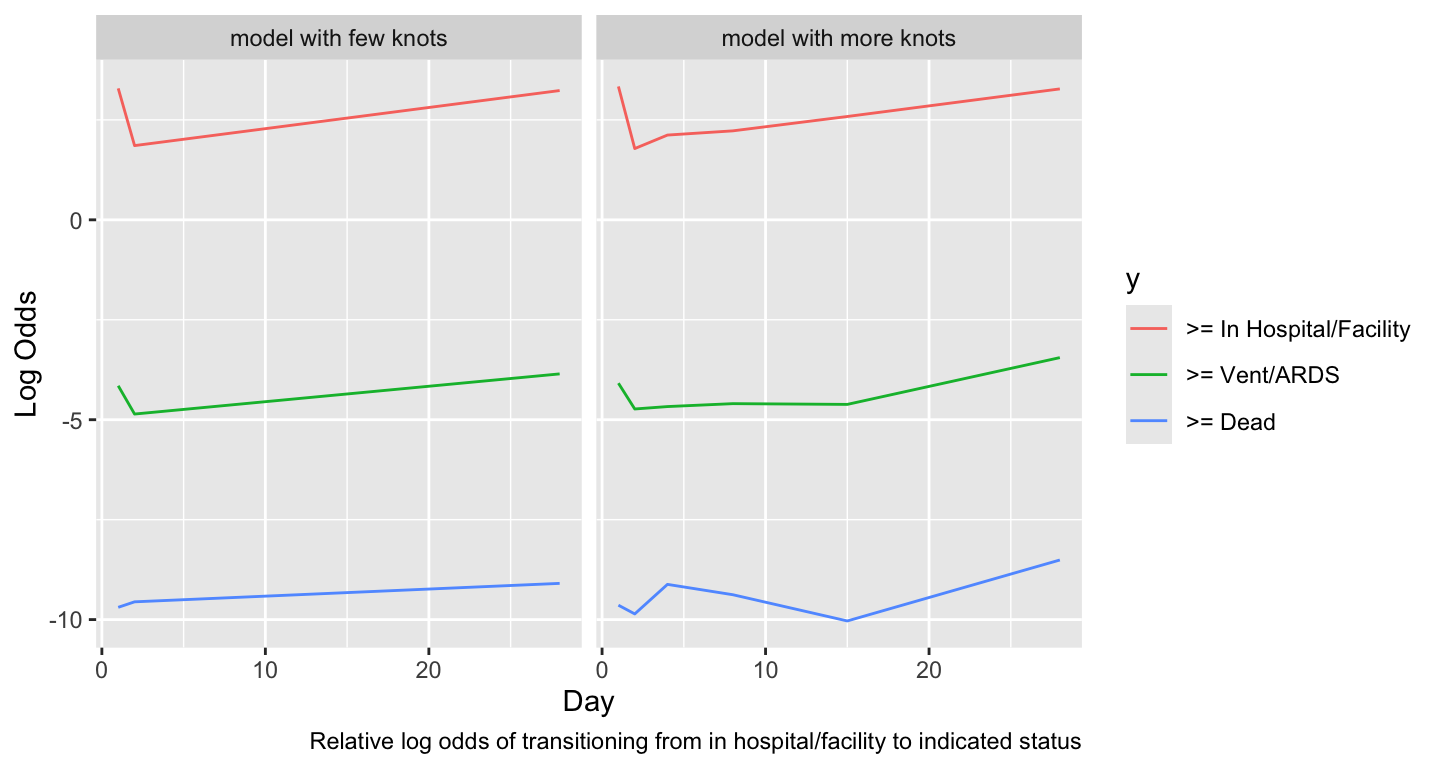Figure 22.3: Estimated time trends in relative log odds of transitions. Variables not shown are set to median/mode and tx=0.

### 22.3.4 Correlation Structure

• The data were simulated under a first-order Markov process so it doesn’t make sense to check correlation pattern assumptions for our model
• When the simulated data were created, the within-patient correlation pattern was checked against the pattern from the fitted model by simulating a large trial from the model fit and comparing correlations in the simulated data to those in the real data
• It showed excellent agreement
• Let’s compute the Spearman $$\rho$$ correlation matrix on the 500 patient dataset and show the matrix from the real data next to it
• Delete day 28 from the new correlation matrix to conform with correlation matrix computed on real data
• Also show correlation matrix from 10,000 patient sample
• Heights of bars are proportional to Spearman $$\rho$$
Code
# Tall and thin -> short and wide data table
w <- dcast(d[, .(id, day, y=as.numeric(y))],
id ~ day, value.var='y')
r <- cor(as.matrix(w[, -1]), use='pairwise.complete.obs',
method='spearman')[-28, -28]
p <- plotCorrM(r, xangle=90)
p[] + theme(legend.position='none') +
labs(caption='Spearman correlation matrix from 500 patient dataset')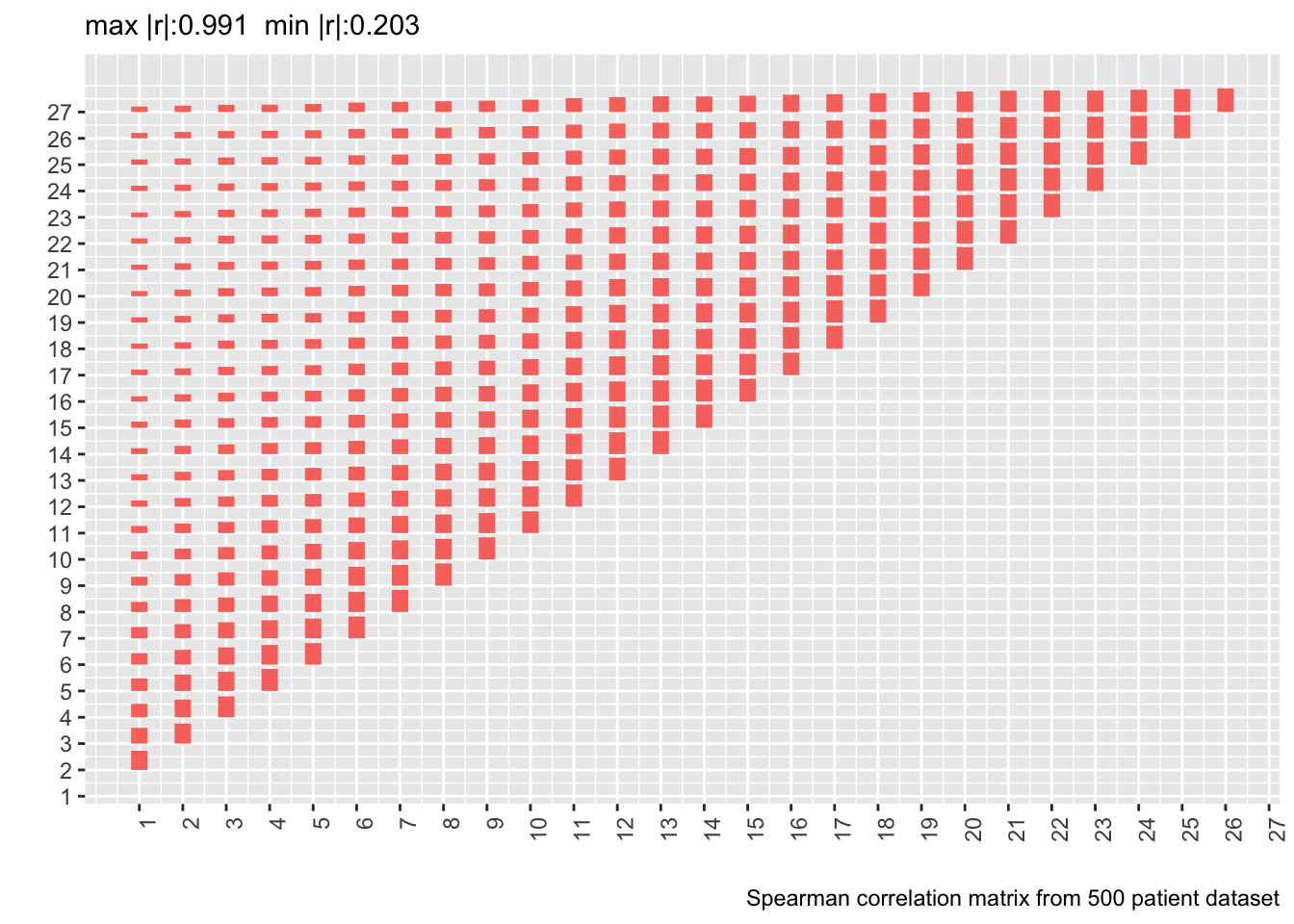Code
vcorr <- readRDS('markov-vcorr.rds')
ra    <- vcorr$r.actual plotCorrM(ra, xangle=90)[] + theme(legend.position='none') + labs(caption='Spearman correlation matrix from actual data')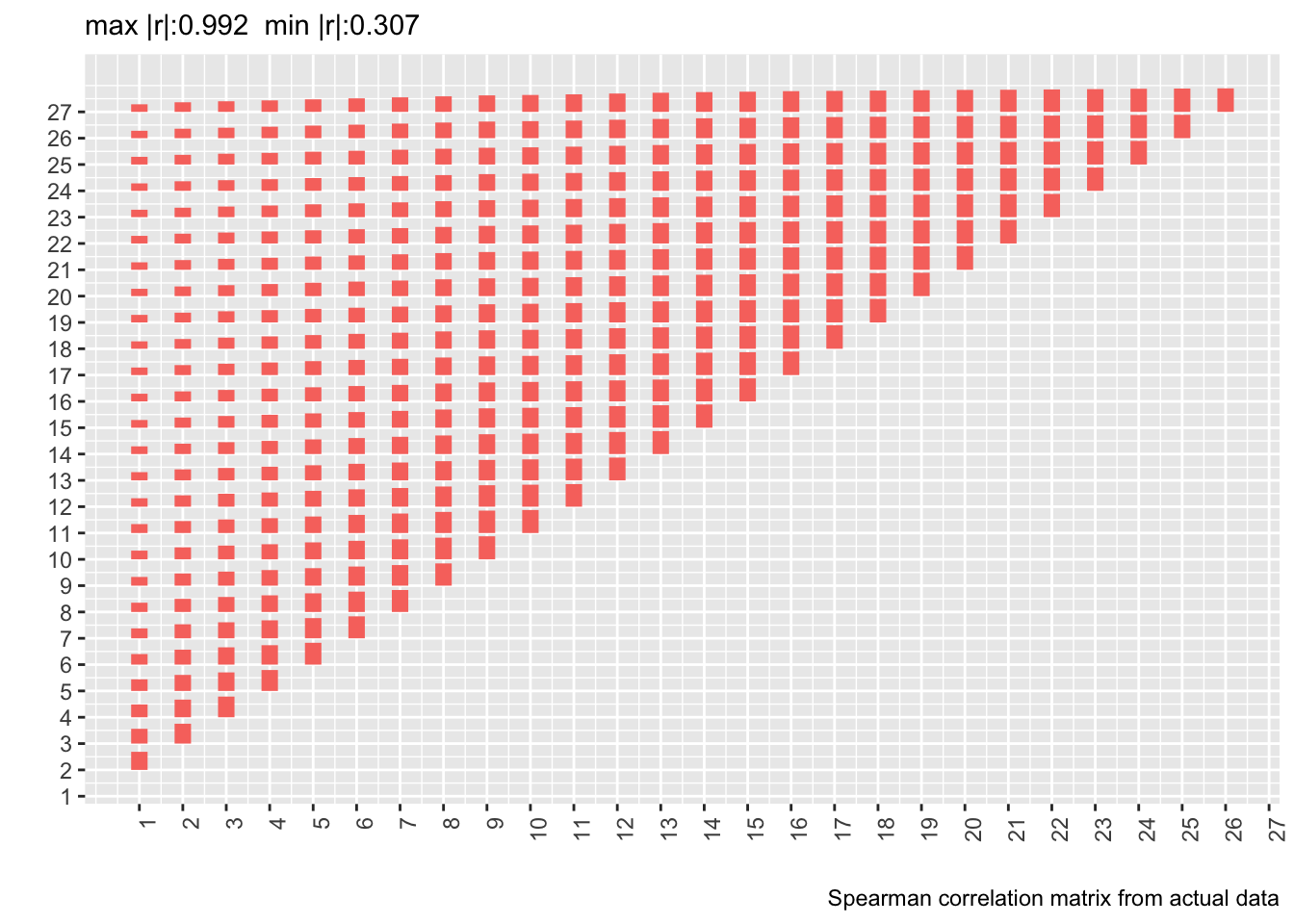Code rc <- vcorr$r.simulated
plotCorrM(rc, xangle=90)[] + theme(legend.position='none') +
labs(caption='Spearman correlation matrix from 10,000 simulated patients')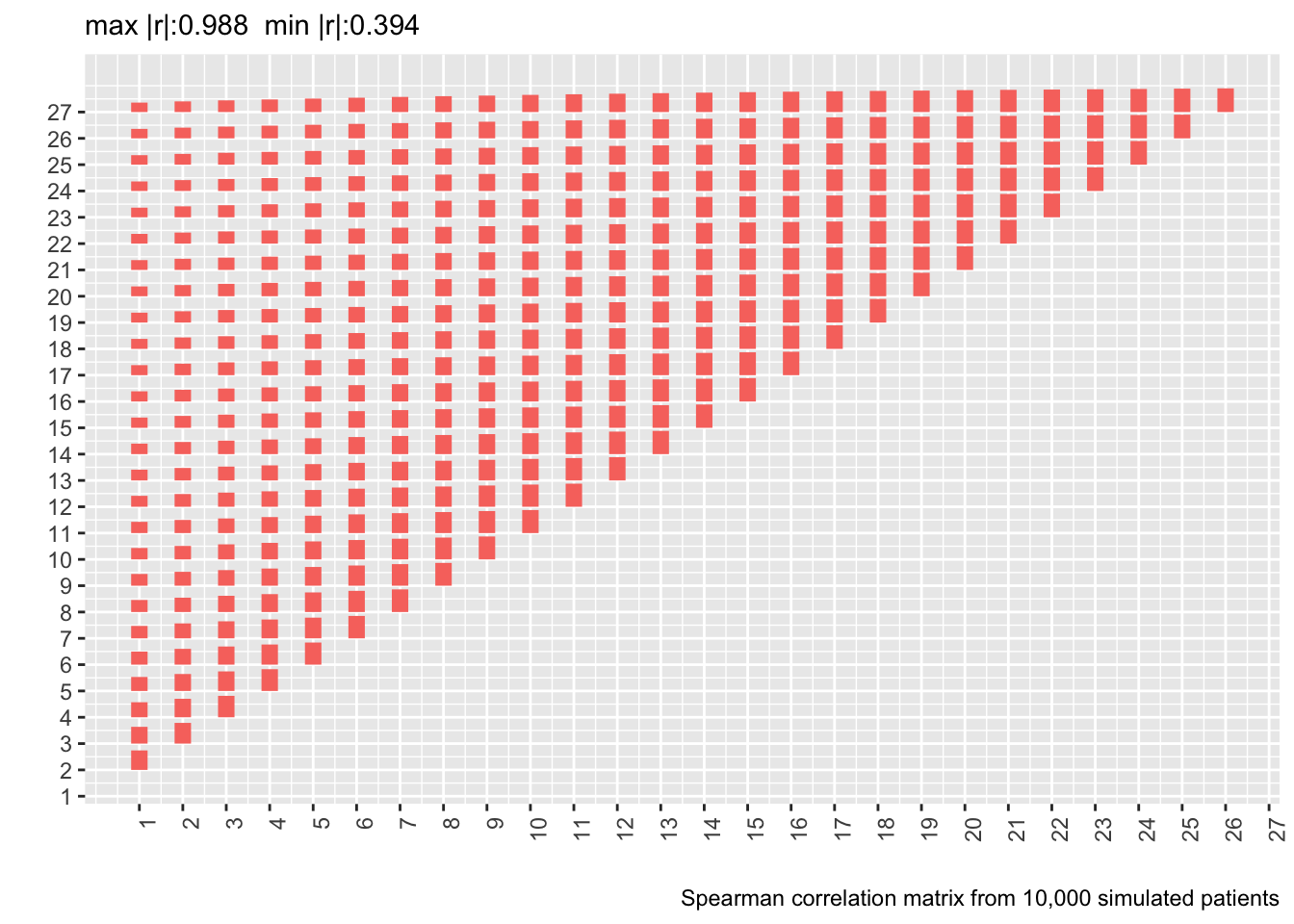• Estimating the whole correlation matrix from 500 patients is noisy
• Compute the mean absolute difference between two correlation matrices (the first on 10,000 simulated patients assuming a first-order Markov process and the second from the real data)
• Compute means of mean absolute differences stratified by the day involved
Code
ad <- abs(rc[-28, -28] - ra)
round(apply(ad, 1, mean), 2)
   1    2    3    4    5    6    7    8    9   10   11   12   13   14   15   16
0.09 0.05 0.05 0.05 0.04 0.03 0.03 0.02 0.02 0.02 0.02 0.02 0.02 0.03 0.02 0.02
17   18   19   20   21   22   23   24   25   26   27
0.02 0.02 0.02 0.02 0.02 0.02 0.02 0.02 0.02 0.02 0.02 

Actual and simulated with-patient correlations agree well except when day 1 is involved.

Code
p[]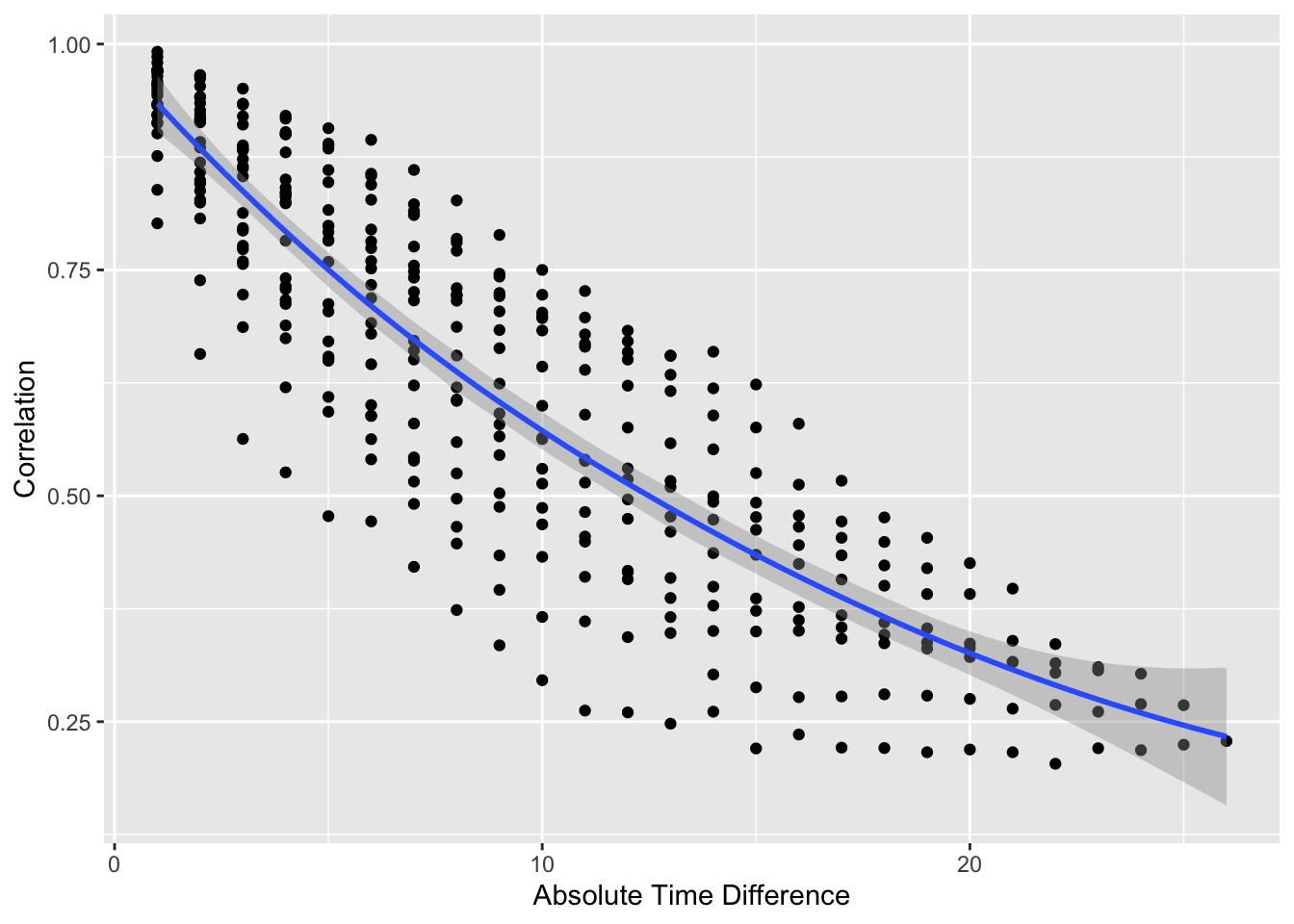Figure 22.4: Variogram-like graph for checking intra-patient correlation structure. $$x$$-axis shows the number of days between two measurements.
• Usual serial correlation declining pattern; outcome status values become less correlated within patient as time gap increases
• Also see non-isotropic pattern: correlations depend also on absolute time, not just gap

#### Formal Goodness of Fit Assessments for Correlation Structure

• Data simulation model $$\rightarrow$$ we already know that the first order Markov process has to fit
• Do two formal assessments to demonstrate how this can be done in general. Both make the correlation structure more versatile.
• Add patient-specific intercepts to see if a compound symmetry structure adds anything to the first-order Markov structure
• Add a dependency on state before last in addition to our model’s dependency on the last state to see if a second-order Markov process fits better

• Bayesian models handle random effects more naturally than frequentist models $$\rightarrow$$ use a Bayesian partial PO first-order Markov model (R rmsb package)
Code
require(rmsb)
options(prType='html')
stanSet()   # sets to use all but one core
seed <- 2   # The following took 15m using 4 cores
b <- blrm(y ~ yprev + lsp(day, 2) + age + sofa + tx + cluster(id),
~ lsp(day, 2), data=d, file='markov-bppo.rds')
stanDx(b)
Iterations: 2000 on each of 4 chains, with 4000 posterior distribution samples saved

For each parameter, n_eff is a crude measure of effective sample size
and Rhat is the potential scale reduction factor on split chains
(at convergence, Rhat=1)
                           n_eff  Rhat
y>=In Hospital/Facility     2399 1.002
y>=Vent/ARDS                1579 1.001
yprev=In Hospital/Facility  2045 1.001
yprev=Vent/ARDS             2544 1.000
day                          755 1.005
day'                        2482 1.000
age                         2725 1.001
sofa                        1007 1.002
tx                          4053 1.001
day:y>=Vent/ARDS             277 1.014
day':y>=Vent/ARDS           3516 1.001
sigmag                      3692 1.000
Code
b

Bayesian Partial Proportional Odds Ordinal Logistic Model

Dirichlet Priors With Concentration Parameter 0.455 for Intercepts

 blrm(formula = y ~ yprev + lsp(day, 2) + age + sofa + tx + cluster(id),
ppo = ~lsp(day, 2), data = d, file = "markov-bppo.rds")


Frequencies of Responses

                 Home In Hospital/Facility            Vent/ARDS
8141                 3887                  913
51

Mixed Calibration/
Discrimination Indexes
Discrimination
Indexes
Rank Discrim.
Indexes
Obs 12992 B 0.028 [0.028, 0.029] g 5.241 [5.012, 5.467] C 0.982 [0.982, 0.982]
Draws 4000 gp 0.453 [0.45, 0.456] Dxy 0.964 [0.963, 0.965]
Chains 4 EV 0.874 [0.863, 0.887]
p 7 v 25.525 [23.636, 27.951]
Cluster on id vp 0.204 [0.201, 0.208]
Clusters 500
σγ 0.3752 [0.0126, 0.6489]
Mean β Median β S.E. Lower Upper Pr(β>0) Symmetry
y≥In Hospital/Facility   -4.6831   -4.7045  0.6465   -5.8992   -3.4127  0.0000  1.08
y≥Vent/ARDS  -12.9337  -12.9341  0.5549  -13.9946  -11.8304  0.0000  1.01
y≥Dead  -19.6333  -19.5992  0.9656  -21.4554  -17.6556  0.0000  0.90
yprev=In Hospital/Facility   8.4580   8.4598  0.2633   7.9483   8.9645  1.0000  1.07
yprev=Vent/ARDS   14.8349   14.8323  0.3094   14.2370   15.4220  1.0000  1.05
day   -1.4770   -1.4592  0.2940   -2.0807   -0.9144  0.0000  0.89
day’   1.5194   1.5052  0.2947   1.0117   2.1757  1.0000  1.12
age   0.0123   0.0122  0.0032   0.0062   0.0183  1.0000  1.01
sofa   0.0751   0.0747  0.0161   0.0430   0.1060  1.0000  1.09
tx   -0.1088   -0.1069  0.0932   -0.2823   0.0745  0.1160  1.00
day:y≥Vent/ARDS   0.7276   0.7241  0.3838   -0.0888   1.4393  0.9730  1.07
day’:y≥Vent/ARDS   -0.7420   -0.7352  0.3876   -1.5099   0.0309  0.0265  0.93
day:y≥Dead   1.6638   1.6422  0.5699   0.5092   2.7334  0.9995  1.03
day’:y≥Dead   -1.6973   -1.6801  0.5766   -2.8004   -0.5556  0.0005  0.97
• Note that blrm parameterizes the partial PO parameters differently than vglm.
• Posterior median of the standard deviation of the random effects $$\sigma_\gamma$$ is 0.22
• This is quite small on the logit scale in which most of the action takes place in $$[-4, 4]$$
• Random intercepts add an inconsequential improvement in the fit, justifying the Markov process’ conditional (on prior state) independence assumption

Second-order Markov Process

• On follow-up days 2-28
• Frequentist partial PO model
Code
# Derive time-before-last states (lag-1 yprev)
h <- d[, yprev2 := shift(yprev), by=id]
h <- h[day > 1, ]
# Fit first-order model ignoring day 1 so can compare to second-order
# We have to make time linear since no day 1 data
f1 <- vglm(ordered(y) ~ yprev + day + age + sofa + tx,
cumulative(reverse=TRUE, parallel=FALSE ~ day), data=h)
f2 <- vglm(ordered(y) ~ yprev + yprev2 + day + age + sofa + tx,
cumulative(reverse=TRUE, parallel=FALSE ~ day), data=h)
lrtest(f2, f1)
Likelihood ratio test

Model 1: ordered(y) ~ yprev + yprev2 + day + age + sofa + tx
Model 2: ordered(y) ~ yprev + day + age + sofa + tx
#Df  LogLik Df  Chisq Pr(>Chisq)
1 37463 -2094.5
2 37465 -2095.0  2 1.1236     0.5702
Code
AIC(f1); AIC(f2)
 4212.051
 4214.928
• First-order model has better fit “for the money” by AIC
• Formal chunk test of second-order terms not impressive

### 22.3.5 Computing Derived Quantities

From the fitted Markov state transition model, compute for one covariate setting and two treatments:

• state occupancy probabilities
• mean time in state
• differences between treatments in mean time in state

To specify covariate setting:

• most common initial state is In Hospital/Facility so use that
• within that category look at relationship between the two covariates
• they have no correlation so use the individual medians
Code
istate <- 'In Hospital/Facility'
w <- d[day == 1 & yprev == istate, ]
w[, cor(age, sofa, method='spearman')]
 0.01651803
Code
x <- w[, lapply(.SD, median), .SDcols=Cs(age, sofa)]
adjto <- x[, paste0('age=', x[, age], '  sofa=', x[, sofa],
'  initial state=', istate)]
# Expand to cover both treatments and initial state
x <- cbind(tx=0:1, yprev=istate, x)
x
   tx                yprev age sofa
1:  0 In Hospital/Facility  56    5
2:  1 In Hospital/Facility  56    5

Compute all SOPs for each treatment. soprobMarkovOrdm is in Hmisc.

Code
S <- z <- NULL
for(Tx in 0:1) {
s <- soprobMarkovOrdm(f, x[tx == Tx, ], times=1:28, ylevels=levels(d$y), absorb='Dead', tvarname='day') S <- rbind(S, cbind(tx=Tx, s)) u <- data.frame(day=as.vector(row(s)), y=as.vector(col(s)), p=as.vector(s)) u$tx <- Tx
z <- rbind(z, u)
}
z$y <- factor(z$y, 1:4, levels(d$y)) revo <- function(z) { z <- as.factor(z) factor(z, levels=rev(levels(as.factor(z)))) } ggplot(z, aes(x=factor(day), y=p, fill=revo(y))) + facet_wrap(~ paste('Treatment', tx), nrow=1) + geom_col() + xlab('Day') + ylab('Probability') + guides(fill=guide_legend(title='Status')) + labs(caption=paste0('Estimated state occupancy probabilities for\n', adjto)) + theme(legend.position='bottom', axis.text.x=element_text(angle=90, hjust=1))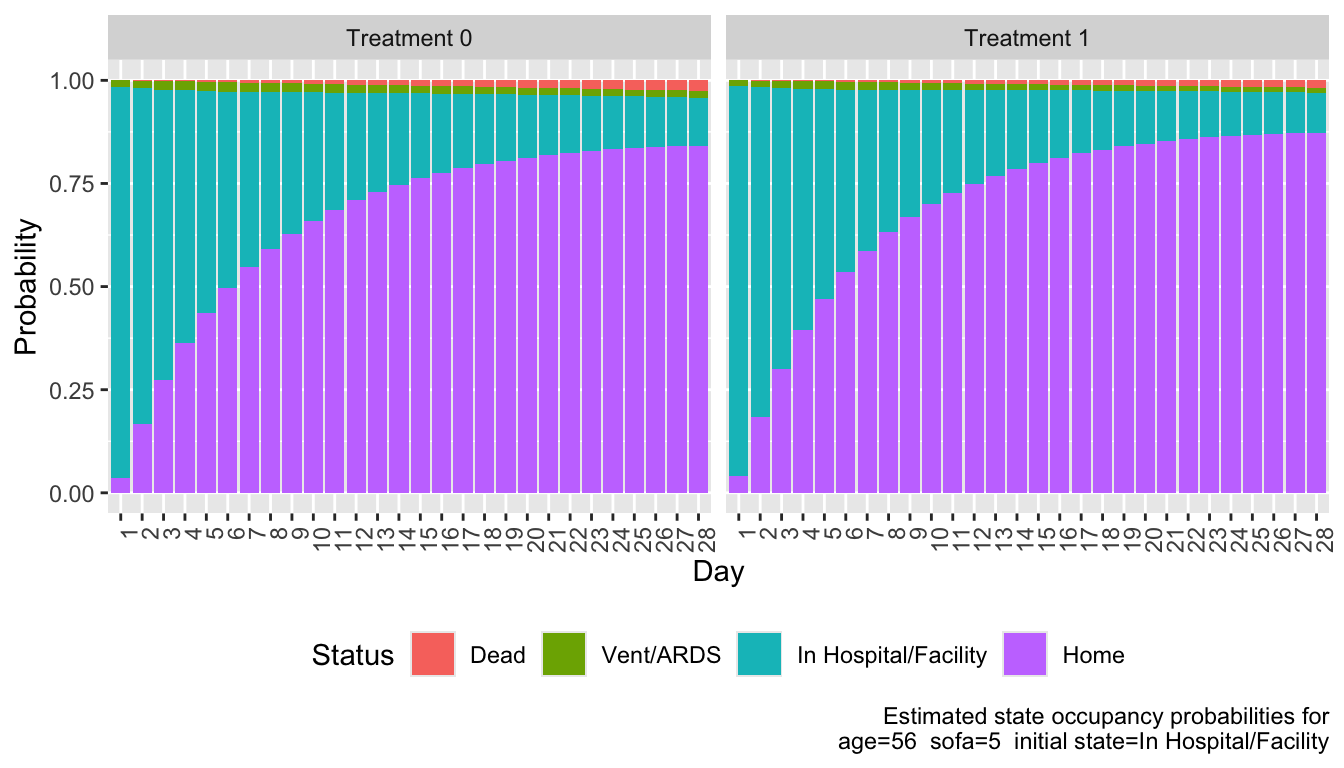Figure 22.5: State occupancy probabilities for each treatment Compute by treatment the mean time unwell (expected number of days not at home). Expected days in state is simply the sum over days of daily probabilities of being in that state. Code mtu <- tapply(1. - S[, 'Home'], S[, 'tx'], sum) dmtu <- diff(mtu) w <- c(mtu, dmtu) names(w) <- c('tx=0', 'tx=1', 'Days Difference') w  tx=0 tx=1 Days Difference 9.5270485 8.5866987 -0.9403498  We estimate that patients on treatment 1 have 3 less days unwell than those on treatment 0 for the given covariate settings. Do a similar calculation for the expected number of days alive out of 28 days (similar to restricted mean survival time. Code mta <- tapply(1. - S[, 'Dead'], S[, 'tx'], sum) w <- c(mta, diff(mta)) names(w) <- c('tx=0', 'tx=1', 'Days Difference') w  tx=0 tx=1 Days Difference 27.65094447 27.74380918 0.09286472  ### 22.3.6 Bootstrap Confidence Interval for Difference in Mean Time Unwell • Need to sample with replacement from patients, not records • code taken from rms package’s bootcov function • sampling patients entails including some patients multiple times and omitting others • save all the record numbers, group them by patient ID, sample from these IDs, then use all the original records whose record numbers correspond to the sampled IDs • Use the basic bootstrap to get 0.95 confidence intervals • Speed up the model fit by having each bootstrap fit use as starting parameter estimates the values from the original data fit Code B <- 500 # number of bootstrap resamples recno <- split(1 : nrow(d), d$id)
npt       <- length(recno)   # 500
startbeta <- coef(f)
seed      <- 3
if(file.exists('markov-boot.rds')) {
betas    <- z$betas diffmean <- z$diffmean
} else {
betas <- diffmean <- numeric(B)
ylev  <- levels(d\$y)
for(i in 1 : B) {
j <- unlist(recno[sample(npt, npt, replace=TRUE)])
g <- vglm(ordered(y) ~ yprev + lsp(day, 2) + age + sofa + tx,
cumulative(reverse=TRUE, parallel=FALSE ~ lsp(day, 2)),
coefstart=startbeta, data=d[j, ])
betas[i] <- coef(g)['tx']
s0 <- soprobMarkovOrdm(g, x[tx == 0, ], times=1:28, ylevels=ylev,
s1 <- soprobMarkovOrdm(g, x[tx == 1, ], times=1:28, ylevels=ylev,
# P(not at home) =  1 - P(home); sum these probs to get E[days]
mtud <- sum(1. - s1[, 'Home']) - sum(1. - s0[, 'Home'])
diffmean[i] <- mtud
}
saveRDS(list(betas=betas, diffmean=diffmean), 'markov-boot.rds', compress='xz')
}

See how bootstrap treatment log ORs relate to differences in days unwell.

Code
ggfreqScatter(betas, diffmean,
xlab='Log OR', ylab='Difference in Mean Days Unwell')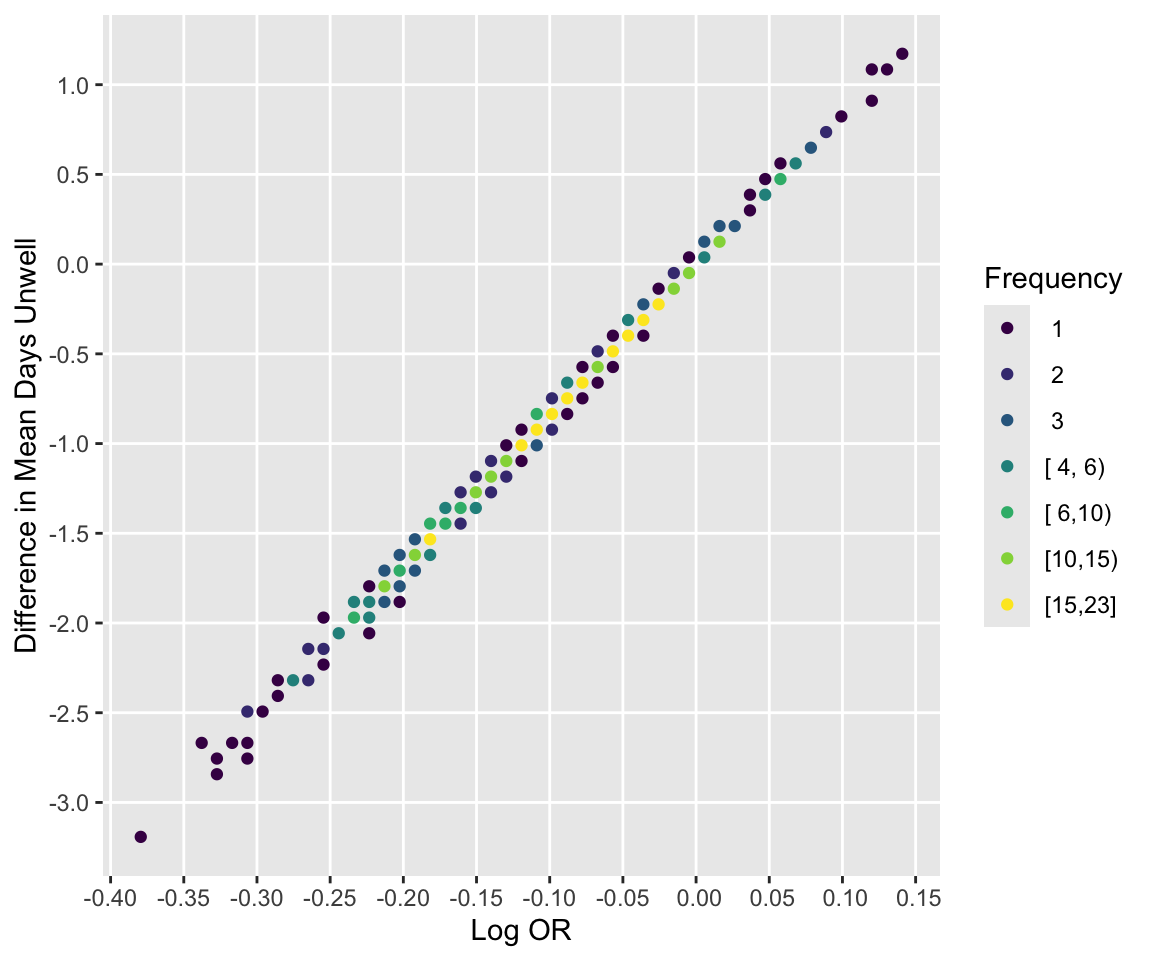Figure 22.6: Relationship between bootstrap log ORs and differences in mean days unwell

Compute basic bootstrap 0.95 confidence interval for OR and differences in mean time

Code
# bootBCa is in the rms package and uses the boot package
clb <- exp(bootBCa(coef(f)['tx'], betas, seed=seed, n=npt, type='basic'))
clm <- bootBCa(dmtu, diffmean, seed=seed, n=npt, type='basic')
a   <- round(c(clb, clm), 3)[c(1,3,2,4)]
data.frame(Quantity=c('OR', 'Difference in mean days unwell'),
Lower=a[1:2], Upper=a[3:4])
                        Quantity  Lower Upper
1                             OR  0.942 1.375
2 Difference in mean days unwell -0.542 2.781

### 22.3.7 Notes on Inference

• Differences between treatments in mean time in state(s) is zero if and only if the treatment OR=1
• note agreement in bootstrap estimates
• will not necessarily be true if PO is relaxed for treatment
• inference about any treatment effect is the same for all covariate settings that do not interact with treatment
• $$\rightarrow p$$-values are the same for the two metrics, and Bayesian posterior probabilities are also identical
• Bayesian posterior probabilities for mean time in state $$> \epsilon$$, for $$\epsilon > 0$$, will vary with covariate settings (sicker patients at baseline have more room to move)